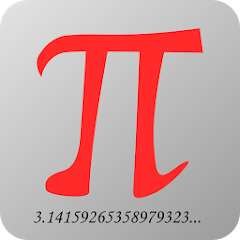# Math - mathematics is easy

3.8
3.72K reviews
500K+Everyone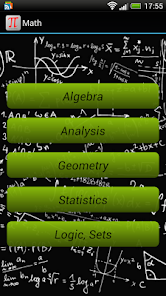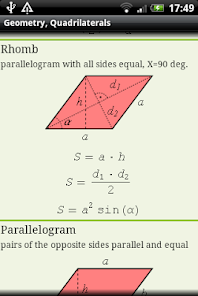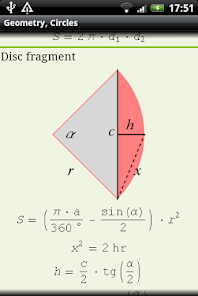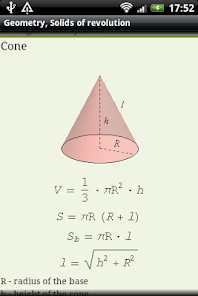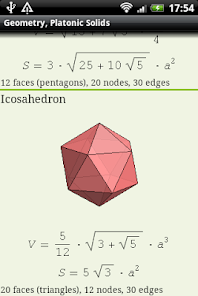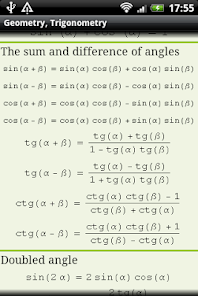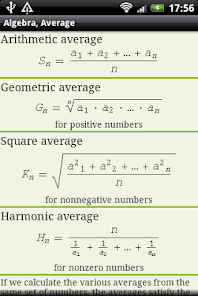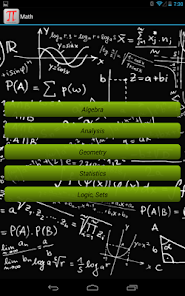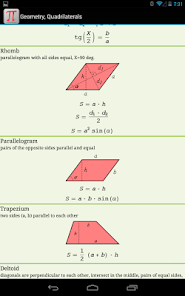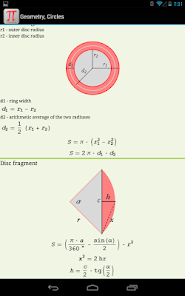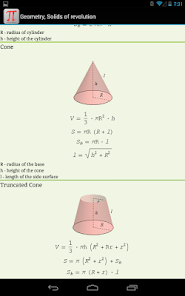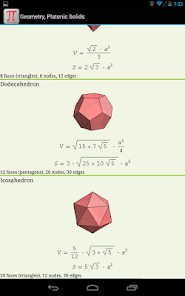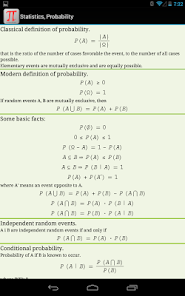Math on the phone!

Now you have all useful math formulas and definitions handy and can always refer to them. There are a lot of drawings (including 3D), explaining all symbols used in formulas.
Range of material - high school.

The content is divided into sections and subsections so that it is easy to find any formula, definition or equation.

Many people have asked about integrals - already are, but here only at the secondary level. Advanced integrals are already available in a twin Matma2 application dedicated to the students.

There is also a paid version of the Math (MathPro), which does not show ads, and includes material from both Math and Math2 apps.

Algebra
- Constants
- Basics
- Exponents
- Averages
- Polynomials
- Logarithms
- Equations
- Equation systems
- Matrices
- Determinants
Analysis
- Sequences
- Limits
- Series
- Integrals properties
- Integrals, basic formulas
Geometry
- Triangles
- Trigonometry
- Polygons
- Circles
- Polyhedrons
- Solids of revolution
- Platonic Solids
Statistics
- Combinatorics
- Probability
Logic, Sets
- Logic

Coming soon: vector calculus, differential calculus, analytic geometry, complex numbers, ... then the next sections.
Updated on
Oct 23, 2013

## Data safetyNo information available

## Ratings and reviews

3.9
3.37K reviews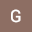George Warren
August 14, 2021
Ugly boring useless app. Rot in hell.
1 person found this review helpful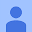June 18, 2014
Whilr this program does not show step by step how to solve by video; the way it is written help you through the process. Now if they had one for physics, chemistry, aviation and other advance mathematics.
12 people found this review helpful